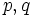# Left residual operator for composition

## Contents

BEWARE! This term is nonstandard and is being used locally within the wiki. [SHOW MORE]

This is a binary subgroup property operator, viz an operator that takes as input two subgroup properties, and outputs one subgroup property

## Definition

### Property-theoretic statement

Suppose$p,q$ are two subgroup properties. The left residual of$p$ by$q$ is the unique subgroup property$r$ such that:$s * q \le p \iff s \le r$.

Here,$*$ denotes the composition operator.

### Statement with symbols

Suppose$p,q$ are two subgroup properties. The left residual of$p$ by$q$ is defined as the subgroup property$r$ as follows:$H$ has property$r$ in$G$ if whenever$G$ is embedded as a subgroup with property$q$ in a group$K$,$H$ has property$p$ in$K$.

## Facts

### Relation with left transiter

Further information: Left transiter

The left transiter of a subgroup property$p$ is defined as the left residual of$p$ by itself.